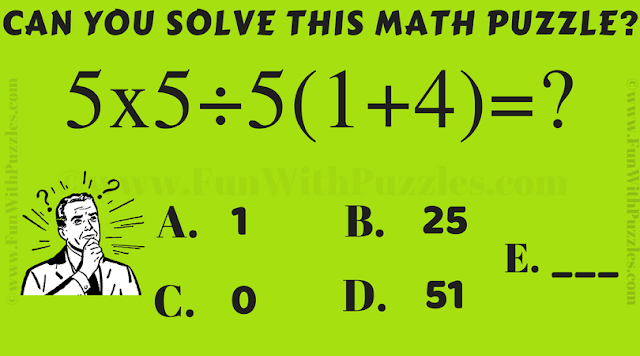This is the quick Math Game Puzzle for school-going teens to test their Mathematical skills. In this Maths Puzzle, you are given a simple Arithmetic equation. Let's see if you can solve this arithmetic equation correctly in your first attempt?Can you solve this Arithmetic Math Game Picture Puzzle?

The answer to this "Arithmetic Math Game Picture Puzzle for Teens", can be viewed by clicking on the button. Please do give your best try before looking at the answer.

Anonymous said...

5x5÷5(1+4)
Mul 5 by 1+4=5+20
5×5÷5+20
Then div 5by5
5×1+20
Then mul 5by1
5+20
Then plus
25

Anonymous said...